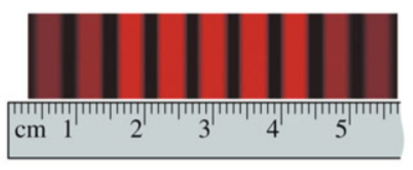# Problem: (Figure 1) shows the light intensity on a screen 2.5 m behind a double slit. The wavelength of the light is 549 nm. What is the spacing between the slits? Express your answer to two significant figures and include the appropriate units.

###### FREE Expert Solution

This problem involves double-slit interference.

The location of the fringes is given by:

$\overline{){\mathbf{d}}{\mathbf{s}}{\mathbf{i}}{\mathbf{n}}{\mathbf{\theta }}{\mathbf{=}}{\mathbf{m}}{\mathbf{\lambda }}}$ d is the slit separation and m is the order.

93% (389 ratings)###### Problem Details
(Figure 1) shows the light intensity on a screen 2.5 m behind a double slit. The wavelength of the light is 549 nm.What is the spacing between the slits? Express your answer to two significant figures and include the appropriate units.